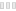# 🔱Technical🔱Question🔱

ForumsGeneral Discussion › 🔱Technical🔱Question🔱
• V҉A҉N҉🍁

Hello there👋

I trying to figure how the 10% income bonus work for a contry directorship.

Suppose you are already director of a state: does the 10% contry income bonus is added at the states income + 10%. Or the contry 10% is only added at the income comming from base with out all other director bonus???

If im not clear lets me know🍺

🔱Astérix🔱🔫

• 🍺Squirrel+Pig🍺

I have always assumed the bonuses were applied in a roll-up fashion, calculating the base first.

• V҉A҉N҉🍁

The first step of director bonus lets me think, he calculate on the base but what about the 3 other?

• 🍺Squirrel+Pig🍺

We'd need the developers to reply unless someone has had the patience to do the math. But I guess I'd assume you add the percentages on starting with the base and then accumulating. I know that when I added a state, I did see that 1% bump from the total of all bases in the state. I assumed it was 1% above the modified income, meaning it already added in 10% and any other 1% bonuses.

• V҉A҉N҉🍁

So the second director ship is calculate like: ((89.56k\$+89.56k\$+78.35k\$)no director bonus)+((59.35k\$+59.35k\$)+10%Of 118.70k\$)=410.10k\$+10% if you are the director of this level 2= 442.11k\$

Each other step should be calculate like the first one... ((()))=... And adding area coming more complex each step...😂

• nͥo͒bͣoͫdy

Math?

• np17036548

Nobody likes math!

• V҉A҉N҉🍁

The first step income bonus up to 199% applied before or afther the director bonus... That is the question...

• V҉A҉N҉🍁

I guess the area bonus (1-199%)its the first bonus to be applied... To that result the 10% (if you are director) is added... For a 209% bonus...

• ☣Alexander☣

But i think 209% is the most you can get. I dont recall seeing bonuses for regions/counties and countries?